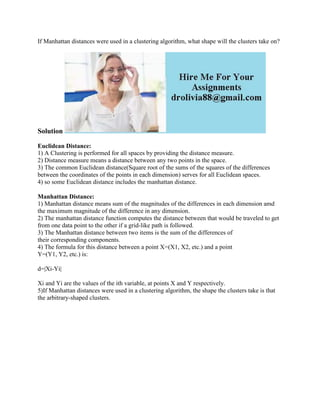Diese Präsentation wurde erfolgreich gemeldet.

# If Manhattan distances were used in a clustering algorithm- what shape.docx

Anzeige
Anzeige
Anzeige
Anzeige
Anzeige
Anzeige
Anzeige
Anzeige
Anzeige
Anzeige
Anzeige×

1 von 2 Anzeige

# If Manhattan distances were used in a clustering algorithm- what shape.docx

If Manhattan distances were used in a clustering algorithm, what shape will the clusters take on?
Solution
Euclidean Distance:
1) A Clustering is performed for all spaces by providing the distance measure.
2) Distance measure means a distance between any two points in the space.
3) The common Euclidean distance(Square root of the sums of the squares of the differences between the coordinates of the points in each dimension) serves for all Euclidean spaces.
4) so some Euclidean distance includes the manhattan distance.
Manhattan Distance:
1) Manhattan distance means sum of the magnitudes of the differences in each dimension amd the maximum magnitude of the difference in any dimension.
2) The manhattan distance function computes the distance between that would be traveled to get from one data point to the other if a grid-like path is followed.
3) The Manhattan distance between two items is the sum of the differences of
their corresponding components.
4) The formula for this distance between a point X=(X1, X2, etc.) and a point
Y=(Y1, Y2, etc.) is:
d=|Xi-Yi|
Xi and Yi are the values of the ith variable, at points X and Y respectively.
5)If Manhattan distances were used in a clustering algorithm, the shape the clusters take is that the arbitrary-shaped clusters.
.

If Manhattan distances were used in a clustering algorithm, what shape will the clusters take on?
Solution
Euclidean Distance:
1) A Clustering is performed for all spaces by providing the distance measure.
2) Distance measure means a distance between any two points in the space.
3) The common Euclidean distance(Square root of the sums of the squares of the differences between the coordinates of the points in each dimension) serves for all Euclidean spaces.
4) so some Euclidean distance includes the manhattan distance.
Manhattan Distance:
1) Manhattan distance means sum of the magnitudes of the differences in each dimension amd the maximum magnitude of the difference in any dimension.
2) The manhattan distance function computes the distance between that would be traveled to get from one data point to the other if a grid-like path is followed.
3) The Manhattan distance between two items is the sum of the differences of
their corresponding components.
4) The formula for this distance between a point X=(X1, X2, etc.) and a point
Y=(Y1, Y2, etc.) is:
d=|Xi-Yi|
Xi and Yi are the values of the ith variable, at points X and Y respectively.
5)If Manhattan distances were used in a clustering algorithm, the shape the clusters take is that the arbitrary-shaped clusters.
.

Anzeige
Anzeige

### If Manhattan distances were used in a clustering algorithm- what shape.docx

1. 1. If Manhattan distances were used in a clustering algorithm, what shape will the clusters take on? Solution Euclidean Distance: 1) A Clustering is performed for all spaces by providing the distance measure. 2) Distance measure means a distance between any two points in the space. 3) The common Euclidean distance(Square root of the sums of the squares of the differences between the coordinates of the points in each dimension) serves for all Euclidean spaces. 4) so some Euclidean distance includes the manhattan distance. Manhattan Distance: 1) Manhattan distance means sum of the magnitudes of the differences in each dimension amd the maximum magnitude of the difference in any dimension. 2) The manhattan distance function computes the distance between that would be traveled to get from one data point to the other if a grid-like path is followed. 3) The Manhattan distance between two items is the sum of the differences of their corresponding components. 4) The formula for this distance between a point X=(X1, X2, etc.) and a point Y=(Y1, Y2, etc.) is: d=|Xi-Yi| Xi and Yi are the values of the ith variable, at points X and Y respectively. 5)If Manhattan distances were used in a clustering algorithm, the shape the clusters take is that the arbitrary-shaped clusters.